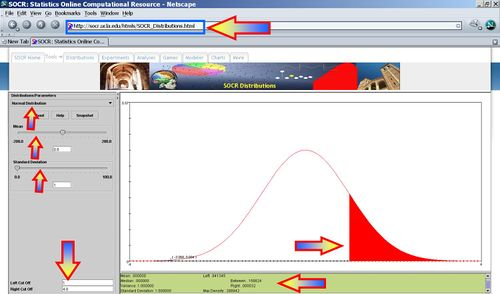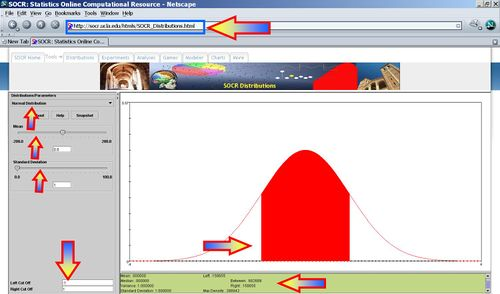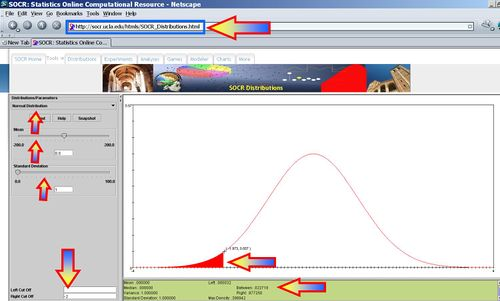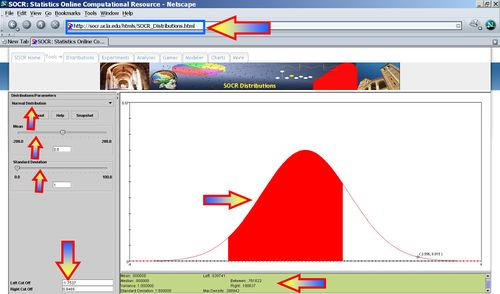# Difference between revisions of "AP Statistics Curriculum 2007 Normal Std"

## General Advance-Placement (AP) Statistics Curriculum - Standard Normal Variables and Experiments

### Standard Normal Distribution

The Standard Normal Cistribution is a continuous distribution where the following exact areas are bound between the Standard Normal Density Function and the x-axis on the symmetric intervals around the origin:

• The area: -1 < x < 1 = 0.8413 - 0.1587 = 0.6826
• The area: -2.0 < x < 2.0 = 0.9772 - 0.0228 = 0.9544
• The area: -3.0 < x < 3.0 = 0.9987 - 0.0013 = 0.9974
Error creating thumbnail: File missing
• Standard Normal density function $$f(x)= {e^{-x^2} \over \sqrt{2 \pi}}.$$
• The Standard Normal distribution is also a special case of the more general normal distribution where the mean is set to zero and a variance to one. The Standard Normal distribution is often called the bell curve because the graph of its probability density resembles a bell.

### Experiments

Suppose we decide to test the state of 100 used batteries. To do that, we connect each battery to a volt-meter by randomly attaching the positive (+) and negative (-) battery terminals to the corresponding volt-meter's connections. Electrical current always flows from + to -, i.e., the current goes in the direction of the voltage drop. Depending upon which way the battery is connected to the volt-meter we can observe positive or negative voltage recordings (voltage is just a difference, which forces current to flow from higher to the lower voltage.) Denote $$X_i$$={measured voltage for battery i} - this is random variable 0 and assume the distribution of all $$X_i$$ is Standard Normal, $$X_i \sim N(0,1)$$. Use the Normal Distribution (with mean=0 and variance=1) in the SOCR Distribution applet to address the following questions. This Distributions help-page may be useful in understanding SOCR Distribution Applet. How many batteries, from the sample of 100, can we expect to have?

• Absolute Voltage > 1? P(X>1) = 0.1586, thus we expect 15-16 batteries to have voltage exceeding 1.• |Absolute Voltage| > 1? P(|X|>1) = 1- 0.682689=0.3173, thus we expect 31-32 batteries to have absolute voltage exceeding 1.• Voltage < -2? P(X<-2) = 0.0227, thus we expect 2-3 batteries to have voltage less than -2.• Voltage <= -2? P(X<=-2) = 0.0227, thus we expect 2-3 batteries to have voltage less than or equal to -2.• -1.7537 < Voltage < 0.8465? P(-1.7537 < X < 0.8465) = 0.761622, thus we expect 76 batteries to have voltage in this range.